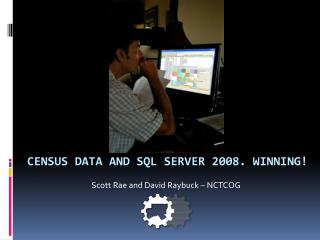Download PresentationCensus DATA And SQL SERVER 2008. winning!

# Census DATA And SQL SERVER 2008. winning! - PowerPoint PPT PresentationDownload Presentation## Census DATA And SQL SERVER 2008. winning!

- - - - - - - - - - - - - - - - - - - - - - - - - - - E N D - - - - - - - - - - - - - - - - - - - - - - - - - - -
##### Presentation Transcript

1. Scott Rae and David Raybuck – NCTCOG Census DATA And SQL SERVER 2008. winning!

2. Census Uses in RIS • Demographic Forecasting • Annual Population Estimates • Small Area Estimates • Storm Impact Modeling • Web Geographic Profiles • Mitigation Planning • Delineation of Geographies • Alternative Futures/Scenarios

3. Census Data Design • Summary File 1 and Public Law • 1990 • 2000 • 2010 Internal Users • Summary File 3 • 2000 Data Bank • American Community Survey • 1 Year • 3 Year • 5 Year Web Users

4. Radius Summaries (emeritus) Circa 2001-2010

5. Census Block Centroids

6. Radius Summary Antiques • ESRI Recordset (Loop Sum) • ESRI Constructed Query (Loop Query Build) • SQL Algebra

7. Selecting by Circle • power((power(( @ptdx - [xcoord]),2) + power((@ptdy -[ycoord]),2)),0.5) < @bufferstring

8. Census Block Centroids

9. Selecting by Polygon select @pointtext = " 0 " select @cn = 0 select @i =1 while @i < @vertexcount begin select @vtx = convert(varchar(16),(select x from #vertex where idx = @i)) select @vty = convert(varchar(16),(select y from #vertex where idx = @i)) select @vtx1 = convert(varchar(16),(select x from #vertex where idx = (@i + 1))) select @vty1 = convert(varchar(16),(select y from #vertex where idx = (@i + 1))) select @pointtext = @pointtext + " + " select @pointtext = @pointtext + "( Case when ((" + @vty + " <= " + @pty + ") and (" + @vty1 + " > " + @pty + ")) or ((" + @vty + " > " + @pty + ") and (" + @vty1 + "<= " + @pty + ")) THEN " select @pointtext = @pointtext + " (Case when " + @ptx + " < (" + @vtx + " + ( ((" + @pty + " - " + @vty + ") / (" + @vty1+ " - " + @vty + " )) * (" + @vtx1 + " - " + @vtx + "))) then 1 else 0 end )" select @pointtext = @pointtext + " else 0 end )" set @i = @i + 1 end exec("insert into #geotemp2 select * from #geotemp where charindex('.5',(" + @pointtext + ") / 2.0) > 0“ )

10. SQL 2008 Spatial • update test2008.dbo.TxGeo_Logrecno set geo9= geography::STPointFromText('POINT(' + STR(Longitude, 20, 16) + ' ' + STR(Latitude, 20, 16) + ')', 4326)

11. SQL 2008 SpatialSelecting by Polygon • set @g = geography::STGeomFromText('POLYGON ((-96 31.4,-97 32.1,-97.1 32.1,-97.5 31.7 ,-97.8 31.7, -96 31.4))', 4326); • SELECT * FROM dbo.txgeo_B WHERE (@g.STIntersects(geog)) = 1

12. Advantages • Speed • Indexing • Expand to Millions of Records • No Geodatabase Overhead • TSQL • Spatial types on Spatial Types

13. Polygon Updating

14. Dynamic Census Image Map

15. Mapping Spatial Data Type SQL 2008 Google Maps/Internet .NET Handler GeoRSS FME Workbench

16. The Logic Inferring numeric polygon values from another polygon layer

17. If I have population by Census block…

18. …what is the population of this triangle?

19. The ArcGIS Way • Calculate original area into source field • ArcToolboxIntersect source with target • Calculate new area into result field • Calculate overlap % (result area / original area) • Multiply this % by value(s) to infer • Summary StatisticsSum (group by Unique ID of target)

20. The SQL Server 2008 Way SELECT Target.UniqueID, SUM(PercentOfTarget * SourceValue) FROM ( SELECT Source.UniqueID, Target.UniqueID, Source.SourceValue, Source.geom.STIntersection(Target.geom).STArea() / Target.geom.STArea() AS PercentOfTarget FROM Target INNER JOIN Source on Source.geom.STIntersects(Target.geom) = 1 ) GROUP BY Target.UniqueID

21. The Grid Optimizing for the web

22. Bing Maps Tile Quadkeys • Length of the key in digits indicates the level of detail (aka zoom level) • Each quadkey starts with the quadkey of the parent grid (the next largest square containing it)

23. Building the Grid --*** ADJUSTABLE VARIABLES ****-- --use the appropriate unit of measurement for the projection you are using (ie feet if state plane): declare @sidelength float = 100 --length of one side of largest grid declare @originX float = 0 --left X coordinate of largest grid cell declare @originY float = 0 --bottom Y coordinate of largest grid cell declare @levels int = 4 --number of tiers to subdivide into 2x2 grids --**** END OF ADJUSTABLE VARIABLES*** declare @leftX float = @originX declare @rightX float = @leftX + @sidelength declare @bottomY float = @originY declare @topY float = @bottomY + @sidelength --create largest grid declare @g geometry set @g = geometry::STGeomFromText('POLYGON((' + str(@leftX, 20, 5) + ' ' + str(@bottomY, 20, 5) + ', ' + str(@rightX, 20, 5) + ' ' + str(@bottomY, 20, 5) + ', ' + str(@rightX, 20, 5) + ' ' + str(@topY, 20, 5) + ', ' + str(@leftX, 20, 5) + ' ' + str(@topY, 20, 5) + ', ' + str(@leftX, 20, 5) + ' ' + str(@bottomY, 20, 5) + '))',2276) Etc…

24. 25.46 million ft2

25. 25.66 million ft2 Error: 0.8%

26. Questions? Scott Rae - srae@nctcog.org David Raybuck - draybuck@nctcog.org